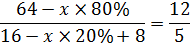# Percentage Quiz For IBPS PO: Part 3

Q.1of a number is 442 less than 3/7 of the same number. What is the 25% of that number?
A. 475.15
B. 625.25
C. 312.50
D. 386.75
E. None of these

Q.2 If the side of a square is decreased by 50%, then find out how much % change in area of that square?
A.75% decrease
B. 50% increase
C. 87.5 decrease
D. 125% increase
E. None of these

Q.3 A milkman has 80 liter mixture of milk and water which has 20% of water. The milkman sold some mixture and add 8 liter of water to the remaining mixture. Now the ratio of the milk and water is 12:5. Find the sold mixture.
A. 5 Liter
B. 20 Liter
C. 16 Liter
D. 4 Liter
E. None of these

Q.4 40% of Aman's annual salary is equal to 15% of Bablu's annual salary. Aman's salary of 3 months is equal to Chintu's salary of 2 months. What is the ratio of Aman, Bablu and Chintu's salary?
A. 9:8:16
B. 5:4:6
C. 6:16:9
D. 7:15:6
E. None of these.

Q.5 In an election, 5% of the total votes are invalid votes. Among 3 candidates, A got 38% of total valid votes, B got 20% of total votes and C got remaining votes. If C won election by 2850 votes then find out total votes?
A. 60000
B. 85000
C. 50000
D. 75000
E. None of these

#### 1. Ans. C

Solution:#### 2. Ans. A.

Solution:=-100+25=75% decrease

#### 3. Ans. B.

Solution:
In 80 liter mixture, Milk=64 liter, Water=16 Liter
Let milkmen sold X liter mixture, then5(64-x×80%) = 12(16-x×20%+8)
5×64 - x×80%×5 = 12×16 - x×20%×12+12×8
320 – x ×400% = 192 - x×240% + 96
320-192-96 = x×400% - x×240%
32 = x×160%
x = 20 Liter

#### 4. Ans. C.

Solution:
Let Aman's annual salary = X, Bablu's annual salary = Y, Chintu's Annual salary = Z
X×40% = Y×15%
Y= X×40/15
X/12×3 = Z/12×2
Z= 6/4×X
X : Y : Z = X : X×40/15 : 6/4×X
= 6:16:9

#### 5. Ans. D.

Solution: